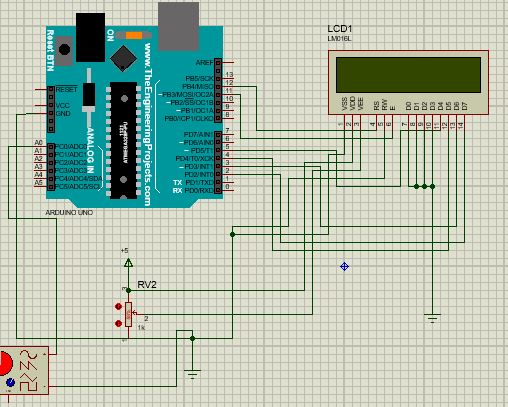# My auto-frequency meter reading is not stable and how to protect my circuit with Zener diode

I want to make an auto-ranging frequency meter to measure the frequency from Hz to some MHz but the circuit could no exceed KHz and above some KHz it become very slow to give the value and the the values it gives are not stable.
And again I'm confused on how to protect the aduino against over-voltage with Zener diode,how should i connect the Zener diode ?
Below is the circuit and the code that i used for this project:

#include <FreqCount.h>
#include <LiquidCrystal.h>
LiquidCrystal lcd(12, 11, 5, 4, 3, 2);
float X;
float f;
float Y;
float Time;
float frequency;
const int pulsePin = A0; // Input signal connected to Pin A0 of Arduino
void setup()
{
pinMode(pulsePin,INPUT);
lcd.begin(16, 2);
lcd.setCursor(0,0);
lcd.print("Frequency meter");
lcd.setCursor(0,1);
lcd.print(" Freq Meter ");
delay(1000);
}
void loop()
{
lcd.clear();
lcd.setCursor(0,0);
lcd.print("F=");
X=pulseIn(pulsePin,HIGH);
Y=pulseIn(pulsePin,LOW);
Time = X+Y;
frequency=1000000/Time;
if(frequency<1000)
{
lcd.clear();
lcd.setCursor(0,0);
lcd.print("F=");
lcd.setCursor(0,1);
lcd.print(frequency);
lcd.print("Hz");
}
else if(frequency<1000000)
{
lcd.setCursor(0,1);
f=frequency/1000.0;
lcd.print(f,3);
lcd.print(" KHz");
}
else if(frequency>=1000000)
{
lcd.setCursor(0,1);
f=frequency/10000.0;
lcd.print(f,6);
lcd.print(" MHz");
}

delay(1000);
}Can someone help me out please,

you used no </> button to insert the code. but I can see you utilize many FLOAT variable. that is bad. And I think you not need second `PulseIn()` - time between two raising flank is that what you want, right?

100kHz is absolute maximum for `pulseIn()`

[Over-voltage protection]u[/u] (I recommend increasing the current-limiting resistor to at least 10K, depending on the driving circuit.)

The documentation says pulseIn() works between 10uS and 3 minutes. So high for 10us and low for 10us is 20us or 50kHz.

And... I'm going to guess that processing the instructions takes some tome so you might not be able process two pulseIn() instructions in a row without extra time in-between.

Consider using an internal counter/timer and an external prescaler such as a divide by 10 (keeps the math simple) ic such as the 4017. Using a 27K resistor in series with the 4017 will give you a lot of protection. If you need it sensitive you can use a fast comparator in front of it.

Clearly expecting the meter to give you readings in MHz without an external circuit is unrealistic. It SHOULD work in principle to give reaings up to around 50kHz.

So can you be a bit more precise about the "some" kHz?

Also be aware your frequency meter will only give correct readings for a rectangular wave that exceeds the Vih and Vil for the input pin.

Thank you sir,actually above 50KHz it will become slow and give incorrect values

In simulation?

Yes

I guess I am getting old, 20KHz was considered the top frequency some humans could hear. You must have a very expensive audio amp and speakers to achieve that frequency. What are you using?

This topic was automatically closed 180 days after the last reply. New replies are no longer allowed.